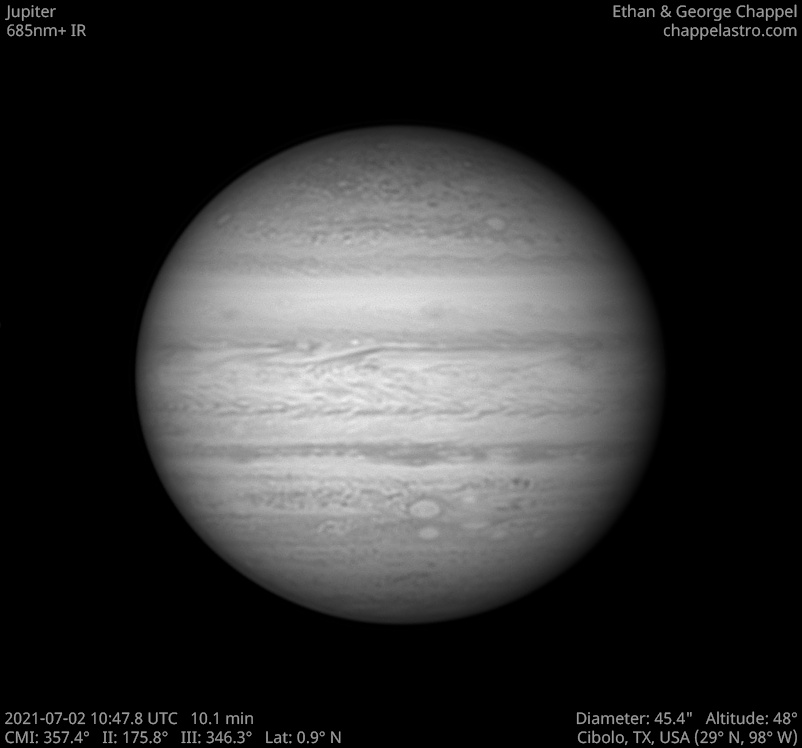# Jupiter 2021-07-02 10:47 UTC

CM1: 357.40°

CM2: 175.80°

CM3: 346.30°

CLat: +0.90°

Description

A beautiful night with excellent seeing July 2, 2021. We were prepared this time and took out the EdgeHD 14 to take high resolution images of Jupiter.

The North Equatorial Belt, or NEB for short, is the most prominent belt in the northern hemisphere of Jupiter. It typically spans from 9 to 17 degrees north of the equator, but the northern edge will occasionally push into the neighboring North Tropical Zone until it reaches 21 degrees north. When this happens, the belt is in an expanded state and usually stays that way for about one Earth year.

When the belt expands northward, two types of storms will emerge from the turbulence: anticyclonic white spots and cyclonic brown barges. Both types of storms will disappear once the NEB recedes southward. One notable exception is known as White Spot Z, which has persisted in the region since 1997.

The NEB is currently receding after an expansion event in 2020. This time, the new northern edge appears to be at 12 degrees north. Northward expansions following these unusually far receding events are typically much more vigorous than typical expansion events, so we may be in for an exciting show anytime from 2023-2025 if this follows the same pattern.

Equipment

ZWO ASI290MM

Celestron EdgeHD 14

Logs
```FireCapture v2.6  Settings
------------------------------------
Observer=Ethan Chappel
Location=Cibolo
Scope=Celestron C14 EdgeHD
Camera=ZWO ASI290MM
Filter=IR685
Profile=Jupiter
Diameter=45.38"
Magnitude=-2.66
CMI=358.1° CMII=176.4° CMIII=347.0°  (during mid of capture)
FocalLength=6750mm (F/18)
Resolution=0.09"
Filename=2021-07-02-1049_5-EC-IR685-Jup.ser
Date=2021_07_02
Start=10_48_33.720
Mid=10_49_33.723
End=10_50_33.726
Start(UT)=10_48_33.720
Mid(UT)=10_49_33.723
End(UT)=10_50_33.726
Duration=120.006s
Date_format=yyyy_MM_dd
Time_format=HH_mm_ss
LT=UT -6h
Frames captured=11993
File type=SER
Binning=no
ROI=784x694
ROI(Offset)=1136x80
FPS (avg.)=99
Shutter=10.00ms
Gain=350 (58%)
SoftwareGain=10 (off)
FPS=100 (off)
USBTraffic=50 (off)
AutoHisto=75 (off)
AutoGain=off
AutoExposure=off
Gamma=50 (off)
HighSpeed=off
Brightness=1 (off)
Histogramm(min)=0
Histogramm(max)=157
Histogramm=61%
Noise(avg.deviation)=4.18
Limit=120 Seconds
Sensor temperature=34.8°C
Focuser position=1480
FireCapture v2.6  Settings
------------------------------------
Observer=Ethan Chappel
Location=Cibolo
Scope=Celestron C14 EdgeHD
Camera=ZWO ASI290MM
Filter=IR685
Profile=Jupiter
Diameter=45.38"
Magnitude=-2.66
CMI=356.2° CMII=174.5° CMIII=345.1°  (during mid of capture)
FocalLength=6650mm (F/18)
Resolution=0.09"
Filename=2021-07-02-1046_4-EC-IR685-Jup.ser
Date=2021_07_02
Start=10_45_26.435
Mid=10_46_26.435
End=10_47_26.435
Start(UT)=10_45_26.435
Mid(UT)=10_46_26.435
End(UT)=10_47_26.435
Duration=120.000s
Date_format=yyyy_MM_dd
Time_format=HH_mm_ss
LT=UT -6h
Frames captured=11992
File type=SER
Binning=no
ROI=784x694
ROI(Offset)=1136x80
FPS (avg.)=99
Shutter=10.00ms
Gain=350 (58%)
SoftwareGain=10 (off)
FPS=100 (off)
USBTraffic=50 (off)
AutoHisto=75 (off)
AutoGain=off
AutoExposure=off
Gamma=50 (off)
HighSpeed=off
Brightness=1 (off)
Histogramm(min)=0
Histogramm(max)=135
Histogramm=52%
Noise(avg.deviation)=3.77
Limit=120 Seconds
Sensor temperature=34.8°C
Focuser position=1480
FireCapture v2.6  Settings
------------------------------------
Observer=Ethan Chappel
Location=Cibolo
Scope=Celestron C14 EdgeHD
Camera=ZWO ASI290MM
Filter=IR685
Profile=Jupiter
Diameter=45.38"
Magnitude=-2.66
CMI=354.4° CMII=172.8° CMIII=343.3°  (during mid of capture)
FocalLength=6700mm (F/18)
Resolution=0.09"
Filename=2021-07-02-1043_5-EC-IR685-Jup.ser
Date=2021_07_02
Start=10_42_31.885
Mid=10_43_31.885
End=10_44_31.885
Start(UT)=10_42_31.885
Mid(UT)=10_43_31.885
End(UT)=10_44_31.885
Duration=120.000s
Date_format=yyyy_MM_dd
Time_format=HH_mm_ss
LT=UT -6h
Frames captured=11993
File type=SER
Binning=no
ROI=784x694
ROI(Offset)=1136x80
FPS (avg.)=99
Shutter=10.00ms
Gain=350 (58%)
SoftwareGain=10 (off)
FPS=100 (off)
USBTraffic=50 (off)
AutoHisto=75 (off)
AutoGain=off
AutoExposure=off
Gamma=50 (off)
HighSpeed=off
Brightness=1 (off)
Histogramm(min)=0
Histogramm(max)=156
Histogramm=61%
Noise(avg.deviation)=4.08
Limit=120 Seconds
Sensor temperature=35.0°C
Focuser position=1480
FireCapture v2.6  Settings
------------------------------------
Observer=Ethan Chappel
Location=Cibolo
Scope=Celestron C14 EdgeHD
Camera=ZWO ASI290MM
Filter=IR685
Profile=Jupiter
Diameter=45.38"
Magnitude=-2.66
CMI=359.3° CMII=177.7° CMIII=348.2°  (during mid of capture)
FocalLength=6700mm (F/18)
Resolution=0.09"
Filename=2021-07-02-1051_6-EC-IR685-Jup.ser
Date=2021_07_02
Start=10_50_39.838
Mid=10_51_39.842
End=10_52_39.846
Start(UT)=10_50_39.838
Mid(UT)=10_51_39.842
End(UT)=10_52_39.846
Duration=120.008s
Date_format=yyyy_MM_dd
Time_format=HH_mm_ss
LT=UT -6h
Frames captured=11993
File type=SER
Binning=no
ROI=784x694
ROI(Offset)=1136x80
FPS (avg.)=99
Shutter=10.00ms
Gain=350 (58%)
SoftwareGain=10 (off)
FPS=100 (off)
USBTraffic=50 (off)
AutoHisto=75 (off)
AutoGain=off
AutoExposure=off
Gamma=50 (off)
HighSpeed=off
Brightness=1 (off)
Histogramm(min)=0
Histogramm(max)=150
Histogramm=58%
Noise(avg.deviation)=4.03
Limit=120 Seconds
Sensor temperature=34.8°C
Focuser position=1480
```